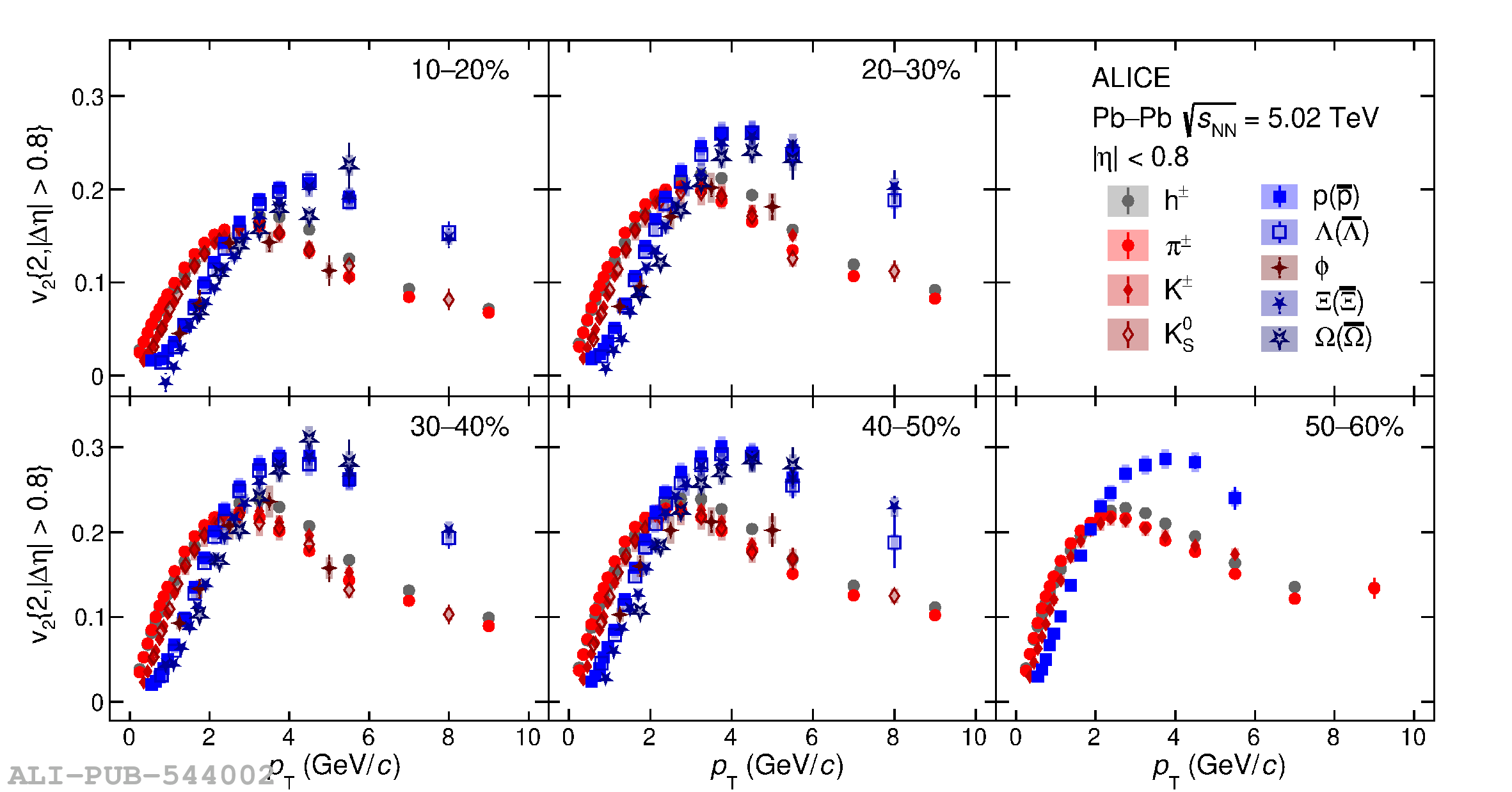# Figure 2

 The \pt-differential $v_2$ measured with two-particle correlations with a pseudorapidity gap of $|\Delta \eta| > 0.8$ for different particle species and centralities in Pb--Pb collisions at $\sqrt{s_{\mathrm{NN}}} = 5.02$ TeV. The vertical error bars and the filled boxes represent statistical and systematic uncertainties, respectively.